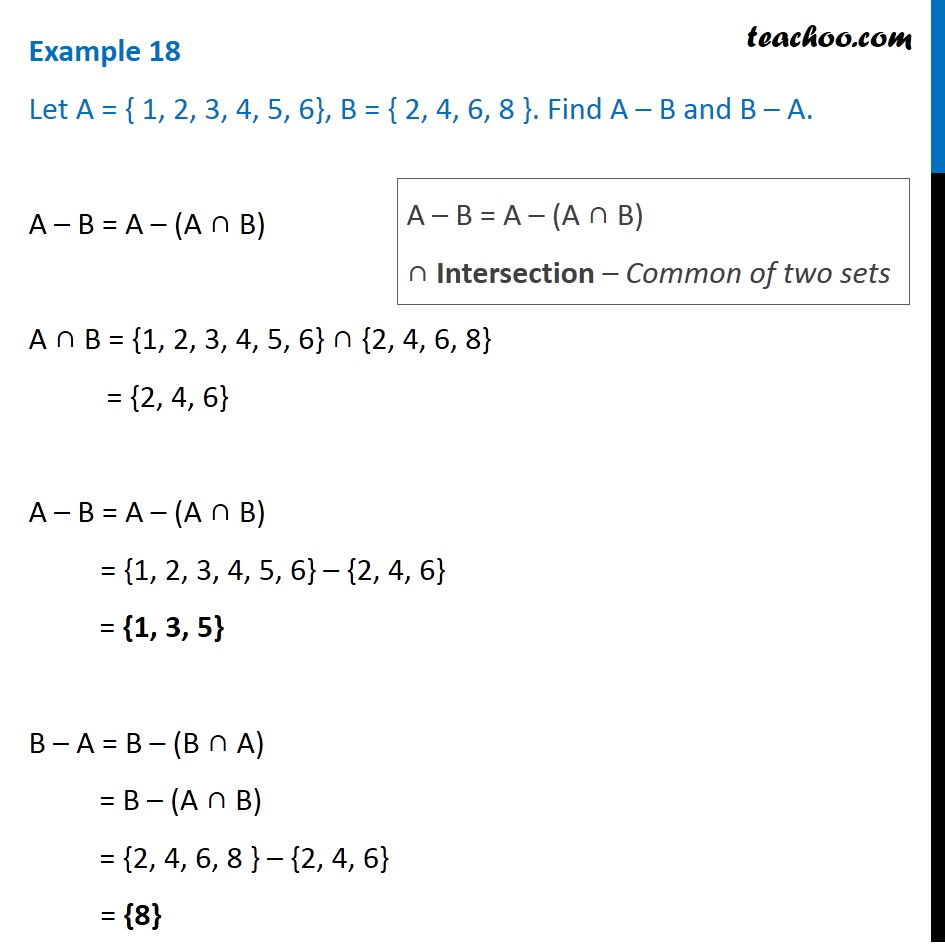1. Chapter 1 Class 11 Sets (Term 1)
2. Concept wise
3. Difference of sets

Transcript

Example 18 Let A = { 1, 2, 3, 4, 5, 6}, B = { 2, 4, 6, 8 }. Find A – B and B – A. A – B = A – (A ∩ B) A ∩ B = {1, 2, 3, 4, 5, 6} ∩ {2, 4, 6, 8} = {2, 4, 6} A – B = A – (A ∩ B) = {1, 2, 3, 4, 5, 6} – {2, 4, 6} = {1, 3, 5} B – A = B – (B ∩ A) = B – (A ∩ B) = {2, 4, 6, 8 } – {2, 4, 6} = {8} A – B = A – (A ∩ B) ∩ Intersection – Common of two sets